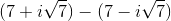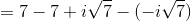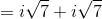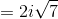## Example Questions

### Example Question #1 : Complex Numbers

From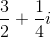, subtract its complex conjugate. What is the difference ?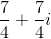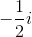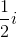Explanation:

The complex conjugate of a complex number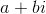is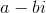, sohas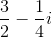as its complex conjugate. Subtract the latter from the former: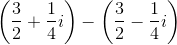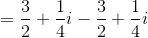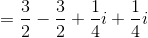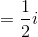### Example Question #2 : Complex Numbers

From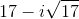, subtract its complex conjugate.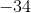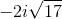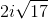Explanation:

The complex conjugate of a complex numberis. Therefore, the complex conjugate ofis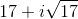; subtract the latter from the former by subtracting real parts and subtracting imaginary parts, as follows: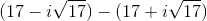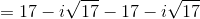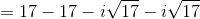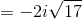### Example Question #3 : Complex Numbers

From, subtract its complex conjugate.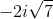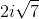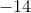Explanation:

The complex conjugate of a complex numberis. Therefore, the complex conjugate of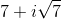is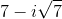; subtract the latter from the former by subtracting real parts and subtracting imaginary parts, as follows: